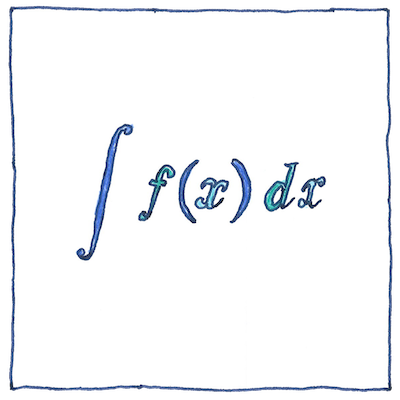# 1671,1684

## The book of science

Tom Sharp

 Isaac Newton, Gottfried Wilhelm Leibniz mathematics

## Calculus

• Newton started with differentiation;
• Leibniz started with integration.
• Newton called it
• the “science of fluents and fluxions”;
• Leibniz called it
• the “calculus.”
• Newton was the first to apply it;
• Leibniz was the first to publish it.
• Newton focused on motion
• and magnitudes;
• Liebiz focused on the tangent
• and on the idea of change.

## Foundations

• Newton’s calculus used infinitesimals
• to solve the problem of planetary motion,
• but infinitesimals were ridiculed
• so Newton gave geometric proofs
• in Principia Mathematica.
• Newton understood the concept of a limit,
• which “can approach so closely
• that their difference
• is less than any given quantity,”
• and proposed limits as an alternative to infinitesimals;
• however, it took another hundred and fifty years
• for mathematicians to provide
• a formal and rigorous definition of calculus.

## Convergence

• Newton knew something about the convergence
• of the infinitesimally small
• and the infinitely large.
• Zeno really messed people up with his paradoxes.
• It took genius to say,
• “You can actually run to the finish line.”
• Zeno said that motion and change are illusions,
• but calculus, the mathematic of motion and change,
• shows us why Zeno was wrong.
• For his first contribution to mathematics
• Newton generalized the binomial theorem
• using infinite series.
• Infinitesimals are arbitrarily small;
• choose any small number;
• we can choose a number that is smaller.
• Infinities are arbitrarily large;
• choose any large number;
• we can choose a number that is larger.
• However, the sum of an infinite series
• isn’t necessarily infinite,
• but can converge to a finite number.

## Steps

• Except for geniuses,
• whom I have known,
• people do learn this stuff
• one step at a time.
• Everything is related
• to everything else,
• pretty much, so you
• can relate to it.
• Should we take little steps
• and risk boring you,
• or big steps
• and risk losing you?

The derivative of a function `f(x) = y` is the slope of the tangent of the curve at the point `(x,y)`. The tangent trigonometric function, defined as the ratio of the sine over the cosine, represents the slope of the circle at the point where the radius touches the circle. Did I get that right?